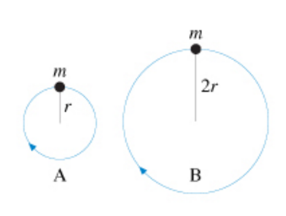# Problem: The figure (figure 1) shows two balls of equal mass moving in vertical circles Is the tension in string A greater than less than, or equal to the tension in string B if the balls travel over the top of the circle with equal angular velocity?a. The tension in string A is greater than the tension in string Bb. The tension in string A is less than the tension in string Bc. The tension in string A is equal to the tension in string B

###### FREE Expert Solution

Angular frequency:

$\overline{){\mathbf{\omega }}{\mathbf{=}}\frac{\mathbf{v}}{\mathbf{r}}}$

Centripetal force:

m and ω are the same for both.###### Problem Details

The figure (figure 1) shows two balls of equal mass moving in vertical circlesIs the tension in string A greater than less than, or equal to the tension in string B if the balls travel over the top of the circle with equal angular velocity?

a. The tension in string A is greater than the tension in string B

b. The tension in string A is less than the tension in string B

c. The tension in string A is equal to the tension in string B